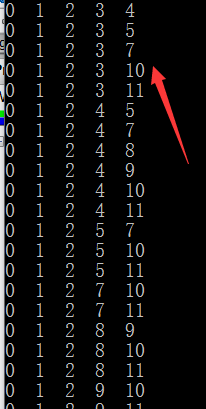2017-03-29 14:01

# 好奇怪一个BUG 各路大神求解 谢谢谢谢！！！

• bug
• 蓝桥杯试题
• 调试

（仅仅连接一个角不算相连）

`````` #include <iostream>
#include <cstdio>
#include <cmath>
#include <queue>
#include <cstring>

using namespace std;

int MP;
int A, B;   //A暂时存选出来的五个数      B保存这个数是不是选过
int sum;
int dx[] = {0,0,1,-1}, dy[] = {1,-1,0,0};
int cut;
struct nod
{
int x, y;
} nd;

queue<nod> qq;

void bfs(int x, int y)      //搜索五个块是不是连着的
{
while(!qq.empty()) qq.pop();

nd.x = x;
nd.y = y;
qq.push(nd);
int m=0;
while(!qq.empty())
{
nd = qq.front();
for(int i=0; i<4; i++)
{
nd = qq.front();
int xx = nd.x+dx[i];
int yy = nd.y+dy[i];
if(xx>=0&&yy>=0&&xx<3&&yy<4&&MP[xx][yy]==1)
{
m++;
MP[xx][yy] = 0;
nd.x = xx;
nd.y = yy;
qq.push(nd);
}
}
qq.pop();
}

if(m==5) sum++;       //如果五个数连着  代表符合要求  sum+1

}

void solve(int cur)             //选出五个没有顺序的数       保证  12345   和 54321 是一种情况 只出现一次
{
if(cur==5)
{
for(int i=0; i<5; i++) printf("%d  ", A[i]);   //调试时输出的每次选出的五个数
cout << endl;
memset(MP, 0, sizeof(MP));
for(int i=0; i<5; i++)
{
MP[ A[i]/4 ][ A[i]%4 ] = 1;
}
bfs(A/4,A%4);
return;
}
for(int i=0; i<12; i++)
{
if(cur==0)
{
if(!B[i])
{
B[i] = 1;
A[cur] = i;
solve(cur+1);
B[i] = 0;
}
}
else
{
if(!B[i]&&i>A[cur-1])
{
B[i] = 1;
A[cur] = i;
solve(cur+1);
B[i] = 0;
}
}
}
}

int main()
{
//   memset(B,0,sizeof(B));
solve(0);
cout << sum <<endl;
return 0;
}

```````````` void bfs(int x, int y)      //搜索五个块是不是连着的
{
while(!qq.empty()) qq.pop();

nd.x = x;
nd.y = y;
qq.push(nd);
int m=0;
while(!qq.empty())
{
nd = qq.front();
for(int i=0; i<4; i++)
{
nd = qq.front();
int xx = nd.x+dx[i];
int yy = nd.y+dy[i];
if(xx>=0&&yy>=0&&xx<3&&yy<4&&MP[xx][yy]==1)
{
m++;
MP[xx][yy] = 0;
//                nd.x = xx;   //这两行
//                nd.y = yy;
qq.push(nd);
}
}
qq.pop();
}

if(m==5) sum++;       //如果五个数连着  代表符合要求  sum+1

}

``````

• 点赞
• 写回答
• 关注问题
• 收藏
• 复制链接分享
• 邀请回答